Previous Article in Journal
Local Productive Systems’ Transitions to Industry 4.0+
Article

# Parameter Estimation of Static/Dynamic Photovoltaic Models Using a Developed Version of Eagle Strategy Gradient-Based Optimizer

1
Department of Electrical Engineering, Faculty of Engineering, Aswan University, Aswan 81542, Egypt
2
Electrical Engineering Department, University of Jaen, 23071 Jaén, Spain
3
K.A.CARE Energy Research and Innovation Center at Riyadh, Riyadh 11451, Saudi Arabia
4
Sustainable Energy Technologies Center, King Saud University, Riyadh 11421, Saudi Arabia
5
Electrical Engineering Department, Mansoura University, Mansoura 35516, Egypt
*
Authors to whom correspondence should be addressed.
Sustainability 2021, 13(23), 13053; https://doi.org/10.3390/su132313053
Received: 27 September 2021 / Revised: 19 November 2021 / Accepted: 20 November 2021 / Published: 25 November 2021

## Abstract

The global trend towards renewable energy sources, especially solar energy, has had a significant impact on the development of scientific research to manufacture high-performance solar cells. The issue of creating a model that simulates a solar module and extracting its parameter is essential in designing an improved and high performance photovoltaic system. However, the nonlinear nature of the photovoltaic cell increases the challenge in creating this model. The application of optimization algorithms to solve this issue is increased and developed rapidly. In this paper, a developed version of eagle strategy GBO with chaotic (ESCGBO) is proposed to enhance the original GBO performance and its search efficiency in solving difficult optimization problems such as this. In the literature, different PV models are presented, including static and dynamic PV models. Firstly, in order to evaluate the effectiveness of the proposed ESCGBO algorithm, it is executed on the 23 benchmark functions and the obtained results using the proposed algorithm are compared with that obtained using three well-known algorithms, including the original GBO algorithm, the equilibrium optimizer (EO) algorithm, and wild horse optimizer (WHO) algorithm. Furthermore, both of original GBO and developed ESCGBO are applied to estimate the parameters of single and double diode as static models, and integral and fractional models as examples for dynamic models. The results in all applications are evaluated and compared with different recent algorithms. The results analysis confirmed the efficiency, accuracy, and robustness of the proposed algorithm compared with the original one or the recent optimization algorithms.

## 1. Introduction

Recently, since the surge in fossil fuel prices, the world’s attention has turned to renewable energy sources, such as wind and solar energy. Solar energy has several advantages beyond resources. These advantages are simple in installation, low maintenance activities, and suitable for different sizes. Although the mentioned advantages of solar energy are still weak regarding high efficiency , increased interest in renewable energy sources has led to an increase in researchers’ interest in developing these systems and increasing their efficiency. Developing an optimal mathematical model that simulates the natural photovoltaic system is one of the biggest challenges for researchers. The difficulty of developing these models is due to the nonlinear properties of solar cells .
Different types of PV models are proposed in the literature. The static model based on the main characteristics of the PV cell as it consists of two semiconductor materials (p-type and n- type) to achieve simple P-N junction characteristics, is considered the base element of the PV system. The PV module is a connection of series and shunt PV cells . The simplest static model is a single diode model (SDM), which has one diode connected with series and shunt resistance [4,5,6,7]. The development in this type of models is based on increasing the accuracy of the models by representing other effects. The double diode model (DDM) is developed to represent the recombination effect in the PV cell. DDM consists of two diodes with one series and one shunt resistance. The total estimated parameters in the DDM are seven parameters, making it more complex than SDM [8,9]. By increasing the number of diodes, other effects can be represented in the model, reflected in increasing the model accuracy; on the other hand, the estimated parameters for the model is also increased, reflected in increasing the model complexity. This idea is illustrated in the three diode model (TDM) [10,11,12]. TDM has three diodes to represent the effect of leakage current and grain boundaries. TDM has nine estimated parameters, so although the model is more accurate, it is considered more complex. The balance between accuracy and complexity is determined according to the application . Although the static model has a wide range of developments in the literature and is more representative to the PV system, the representation of the load connection, variation, and switching are missed. The dynamic model has been proposed in the literature to overcome this problem by representing the load connection in the model [14,15,16,17]. The dynamic models proposed in the literature are the integer and fractional dynamic models. The integer dynamic model is considered the more popular dynamic model and the fractional model has been developed to increase the accuracy of the integer model . All these models have different parameters. These parameters control the model output (dependent variable) based on the model input (independent variable). The well estimation of these parameters has a direct effect in the model accuracy, for which many researchers have proposed to discuss. Parameters estimation using optimization algorithms has been discussed by several researchers. A review about the applied optimization techniques to estimate the parameters of the PV models is proposed in . The numerical/analytical methods are applied for the optimization problems but these methods achieve low accuracy solutions. Population based algorithms are applied widely for these problems as it is simple in application with more accurate results. Population based algorithms are too many to discuss in this paper, but one example includes the recently proposed Whippy Harris Hawks Optimization Algorithm (WHHO) . WHHO is an enhancement for HHO proposed by . HHO is inspired by a group of hawks in catching their prey. WHHO improves the weak in HHO by reducing the local optima problem occurrence besides increasing the algorithm search speed. This meta-heuristic method is a derivative-free optimization method. It has no restrictions on the objective function and has a great advantage in solving multimodal problems. Therefore, it is employed to solve many optimization problems, such as the high performance SPR sensor design , solar-based DG allocation , optimal reactive power dispatch , and parameter estimation of photovoltaic [25,26,27]. One recent application of meta-heuristic algorithm for PV parameters estimation is the application of wild horse optimizer (WHO) for parameter estimation of modified double-diode and triple-diode photovoltaic models proposed in . Another interesting work is the application of an improved bald eagle search algorithm for parameter estimation of different photovoltaic models proposed in . The improved algorithm has been tested through three static PV models SDM, DDM, and TDM. Moreover, three modified static PV models MSDM, MDDM, and MTDM. The study in [28,29] is interesting due to it using recent algorithms with modified static models, but the dynamic PV models are missed.
The GBO algorithm is considered a metaheuristic optimization algorithm inspired by gradient descent and the Newton method . GBO has advantage of rapid convergence due to gradient search rules and also reducing probability of escaping from local optima. ESCGBO is a modified version of GBO proposed in this paper. The ESCGBO technique is an enhancement applied to the GBO using eagle strategy (ES) with chaotic method to enhance the balance between global search or exploration and local search or exploitation and then increasing the original algorithm performance. The ES has been used in several studies to improve the performance of the conventional algorithms, such as an improved particle swarm optimization (PSO) algorithm [31,32], adaptive Nelder–Mead simplex algorithm , and JAYA algorithm and Nelder–Mead simplex method , eagle strategy with flower algorithm , while chaotic maps were also used to enhance several optimization algorithms, such as developing chaotic HHO algorithm , chaotic artificial ecosystem-based optimization (CAEO) algorithm , chaotic JAYA algorithm , and chaotic salp swarm algorithm (CSSA) . In this article, the ESCGBO algorithm is proposed and applied to estimate different PV static and dynamic PV models. The results from all applications are analyzed and evaluated.
• Proposing a developed version of GBO algorithm based on the eagle strategy with the chaotic method to enhance the performance and avoiding the local optima.
• Applying the conventional GBO and ESCGBO as well as other well-known optimization algorithms for parameter estimation of different PV models, such as static and dynamic PV models.
• The results confirm that the proposed ESCGBO has the capability to enhance the performance and increase the effectiveness of the conventional GBO and improve the convergence rate
The remainder of this paper is arranged as follow: Section 2 presents the static and dynamic PV models. ESCGBO is proposed in Section 3. The results and analysis is discussed in Section 4. Section 5 presents the conclusion.

## 2. PV Static and Dynamic Models

PV models should accurately describe the characteristics of the PV systems for different types of PV models proposed in literature in order to achieve this target. In this section, the most popular static and dynamic models are discussed.

#### 2.1. Static SDM and DDM

SDM has one diode connected with series resistance and parallel resistance to the photo generated current, represented with current source connected parallel with the diode. SDM has five parameters; therefore, it is considered the simplest model. Consider x is a vector of model parameters x = (x1, x2, x3, x4, x5) equivalent to (Rs, Rsh, Iph, Is, ɳ). The SDM equivalent circuit is shown in Figure 1 and represented by Equations (1) and (2). The DDM is developed to represent the effect of recombination in the PV cell, and this is achieved by adding a second diode to the SDM circuit as shown in Figure 2. The DDM has seven parameters x = (x1, x2, x3, x4, x5, x6, x7) equivalent to (Rs, Rsh, Iph, Is1, Is2, ɳ1, ɳ2). The DDM is described by Equations (3) and (4). The objective function for SDM and DDM is described by Equations (5) and (6) respectively.
$I = I p h − I D − I s h$
$I = I p h − I s [ exp ( q ( V + R s ∗ I ) η ∗ K ∗ T ) − 1 ] − ( V + R s ∗ I ) R s h$
$I = I p h − I D 1 − I D 2 − I s h$
$I = I p h − I s 1 [ exp ( q ( V + R s ∗ I ) η 1 ∗ K ∗ T ) − 1 ] − I s 2 [ exp ( q ( V + R s ∗ I ) η 2 ∗ K ∗ T ) − 1 ] − ( V + R s ∗ I ) R s h$
$f D D ( V , I , X ) = I − X 3 + X 4 [ exp ( q ( V + X 1 ∗ I ) X 5 ∗ K ∗ T ) − 1 ] + ( V + X 1 ∗ I ) X 2$
$f D D ( V , I , X ) = I − X 3 + X 4 [ exp ( q ( V + X 1 ∗ I ) X 6 ∗ K ∗ T ) − 1 ] + X 5 [ exp ( q ( V + X 1 ∗ I ) X 7 ∗ K ∗ T ) − 1 ] + ( V + X 1 ∗ I ) X 2$

#### 2.2. Dynamic PV Model

The integral and fractional dynamic PV models are two popular dynamic models, and are selected to be discussed here.
The integral dynamic model (IOM) is a second order model consists of constant voltage source $V o c$ and series resistance $R S$ to represent the static model as shown in Figure 3. The dynamic part is represented by capacitor $C$ for junction capacitance and resistance $R c$ for conductance. The connected cables inductance is represented by the coil inductance. The load is represented by.The total number of unknown parameters are three parameters (), the IOM is represented by Equations (7) and (8).
$i L ( s ) = V o c s a 11 ( s + b 1 ) + b 2 ( s − a 11 ) ( s − a 22 ) ( s − a 11 ) − a 12 a 21$
The fractional order model (FOM) is developed to represent fractional capacitor in case of low values of $R c$ due real frequency dependence on fractional capacitance impedance, as shown in Figure 4. The fractional order of capacitance and inductance are represented by α and β, respectively. The total number of FOM is five parameters (), the IOM is represented by Equations (9) and (10).

## 3. Optimization Methodology

This section describes the basic variant of the gradient-based optimizer (GBO). After, the process of the proposed EGCGBO algorithm is presented.

#### 3.1. Original Gradient-Based Optimizer (GBO) Algorithm

The GBO algorithm is a proposed metaheuristic optimization algorithm, which is presented in reference . This algorithm uses the Newton’s gradient-based method to explore the whole search space. This algorithm uses two main machinists, namely gradient search rule (GSR) and local escaping operator (LEO) to achieve the best solution.

#### 3.1.1. GBO Initialization

Like many algorithms, the GBO comprises $N$ vector (members of populations) in the D-dimensional search space. The initial population is randomly generated by the following equation :
$X n = X m i n + r a n d ( 0 , 1 ) × ( X m a x − X m i n )$
where $X n$ refers to the nth vector, are the limits of the solution space in each problem and denotes a random number defined in the range of [0, 1].

#### 3.1.2. Gradient Search Rule (GSR)

In the GBO algorithm, GSR is based on the gradient-based method where the aim of using the GSR is exploration tendency improvement and increasing the convergence rate. Therefore, the new position $X n + 1$ is defined as:
$X n + 1 = X n − 2 ∆ x × f ( X n ) f ( X n + ∆ x ) − f ( X n − ∆ x )$
The Equation (12) will be adjusted to include the population-based search theory, which is presented by Equation (13).
where is a random number with a normal distribution, denote the worst and best solutions obtained during the process of optimization, $ε$ denotes a small number within the interval [0, 0.1], and $∆ x$ refers to the change in position at each iteration. From the previous equations, the GSR can be defined as:
where $ρ 1$ denotes the randomly generated parameter and it calculated from the following equation:
$ρ 1 = ( 2 × r a n d × α ) − α$
$β = β m i n + ( β m i n − β m i n ) ( 1 − ( m M ) 3 ) 2$
where $α$ represents a sine function for the transference from exploration to exploitation, $β m i n$ and $β m a x$ are constant values 0.2 and 1.2, respectively, $m$ is the current number of iterations, and $M$ represents the total number of iterations. The change $∆ x$ between the best solution $x b e s t$ and a randomly chosen position $x r 1 m$ is given by:
$∆ x = r a n d ( 1 : N ) × | s t e p |$
$s t e p = ( x b e s t − x r 1 m ) + δ 2$
$δ = 2 × r a n d × ( | x r 1 m + x r 2 m + x r 3 m + x r 4 m 4 | − x n m )$
where $r a n d ( 1 : N )$ denotes a random vector with N dimensions, ) are different integers randomly chosen from [1, N], $s t e p$ denotes a step size. The new position $X n + 1$ represents an updated version based on the GSR as follows:
$X n + 1 = X n − G S R$
The direction of movement (DM) is added for better exploitation of the nearby area of $X n$ which can be calculated as:
$D M = r a n d × ρ 2 × ( x b e s t − x n )$
$ρ 2 = ( 2 × r a n d × α ) − α$
Therefore, the new position $X 1 n m$ is calculated from the following equation after taking the GSR and DM into consideration:
$X 1 n m = x n m − GSR + DM$
$X 1 n m = x n m − r a n d n × ρ 1 × 2 Δ x × x n m ( x worst − x b e s t + ε ) + r a n d × ρ 2 × ( x b e s t − x n m )$
The GBO used another position to increase the local search by putting the best-so-far solution ($x best$) rather than the position $x n m$. The new position ($X 2 n m$) is calculated as follows:
$X 2 n m = x best − r a n d n × ρ 1 × 2 Δ x × x n m ( y p n m − y q n m + ε ) + r a n d × ρ 2 × ( x r 1 m − x r 2 m )$
where
$y p n = r a n d × ( [ z n + 1 + x n ] 2 + r a n d × ∆ x )$
$y q n = r a n d × ( [ z n + 1 + x n ] 2 − r a n d × ∆ x )$
According to the positions $X 1 n m$,, and the current position ($X n m )$, the new position at the next iteration ($x n m + 1$) is defined as:
$x n m + 1 = r a × ( r b × X 1 n m + ( 1 − r b ) × X 2 n m ) + ( 1 − r a ) × X 3 n m$
$X 3 n m = X n m − ρ 1 × ( X 2 n m − X 1 n m )$

#### 3.1.3. Local Escaping Operator (LEO)

The LEO is applied to enhance the performance of the GBO algorithm and to escape the local solutions for solving the complicated problems. The LEO generates an appropriate solution ($X L E O m$) by using several solutions, which include $x best$, the solutions $X 1 n m$, and $X 2 n m$, two random solutions $x r 1 m$ and $x r 2 m$, and a new randomly generated solution ($x k m$). The solution $X L E O m$ is formulated as:
where $f 1$ denotes a uniform distributed random number in the range of [−1, 1], $f 2$ is a random number from a normal distribution with a mean of 0 and a standard deviation of 1, $p r$ is the probability, and $u 1$, $u 2$, and $u 3$ are random values generated as follows:
where represents a random number in the range of [0, 1], and $μ 1$ refers to a number in the range of [0, 1]. The above equations are simply explained as follows:
$u 1 = L 1 × 2 × r a n d + ( 1 − L 1 )$
$u 2 = L 1 × r a n d + ( 1 − L 1 )$
$u 3 = L 1 × r a n d + ( 1 − L 1 )$
where $L 1$ denotes a binary parameter with a value of 0 or 1. If parameter $μ 1$ < 0.5, the value of $L 1$ = 1, otherwise, = 0. The solution $x k m$ is generated as follows:
where $x r a n d$ is a random generated solution, $x p m$ is a randomly chosen solution of the population (]), and $μ 2$ represents a random number in the range of [0, 1]. Equation (38) is simplified as:
$x k m = L 2 × x p m + ( 1 − L 2 ) × x r a n d$
where $L 2$ denotes a binary parameter with a value of 0 or 1. If $μ 2$ < 0.5, the value of $L 2$ = 1, otherwise, $L 2$ = 0.

#### 3.2.1. Eagle Strategy

The eagle strategy is proposed for solving real-world optimization problems that is developed by Yang et al. . It is inspired by the foraging behavior of eagles that fly randomly in analogy to the Levy flights. It is the two-stage method: global search randomization stage and an intensive local search . The first stage aims mainly to investigate the search space globally and rapidly obtain a promising solution, while the target of the second stage is to obtain the optimal solution through making an intensive local search based on the achieved solution in the first stage. The benefit of this strategy is that there is no limit to the kinds of techniques or algorithms used in each stage. Any technique that is able to achieve better results in a flexible way could be used in any stage .
During the iteration of the proposed technique, the new candidate solution is generated by Levy flight as follows:
$X n + 1 = X n − γ ( X n − X b e s t ) ⨁ L e v y ( λ ) = X n + 0.01 u | v | 1 / λ ( X n − X b e s t )$
where $γ$ is the step scaling size, the $⨁$ refers to the process of element-wise multiplications, $λ$ is the Levy flight exponent, while u and v can be expressed as:
The standard deviations $σ u$ and $σ v$ are explained as:
where $Γ$ is the Gamma function.

#### 3.2.2. Chaotic Maps

Chaotic systems are deterministic systems that present unpredictable conduct, whose action is complex and similar to that of randomness . In , chaos-based exploration rate to enhance the performance of three well-known optimization algorithms was proposed. Based on this proposal, the real random number ($v$) in Equation (41) was replaced by a chaotic number in the eagle strategy. This modification makes the value of $v$ linearly decreased from 2 to 0 throughout the course of iterations. The steps of the proposed ESCGBO are presented in the flowchart illustrated in Figure 5.

## 4. Results

#### 4.1. Performance of the Proposed ESCGBO Algorithm

In this subsection, the effective and performance of the proposed ESCGBO technique were evaluated on several benchmark functions, including statistical measurements, such as best values, mean values, worst values, and standard deviation (STD) for the solutions obtained by the ESCGBO technique, the original GBO algorithm as well as the two recent optimization algorithms, including the equilibrium optimizer (EO) algorithm  and wild horse optimizer (WHO) algorithm . The results obtained with the proposed ESCGBO algorithm were compared with these well-known optimization algorithms. All mentioned techniques were executed for the maximum number of the iterations’ function of 500 and population size of 50 for 20 independent runs using Matlab R2016a’working on Windows 8.1, 64bit. All computations were performed on a Core i5-4210U [email protected] 2.40 GHz of speed, and 8 GB of RAM. Figure 6 shows the qualitative metrics on F1, F3, F4, F5, F8, F12, F15, and F18, including 2D views of the functions, search history, average fitness history, and convergence characteristics curve using the proposed ESCGBO algorithm.
Table 1, Table 2 and Table 3 present the statistical results of the proposed ESCGBO algorithm and other recent techniques when applied for unimodal benchmark functions, multimodal benchmark functions, and composite benchmark functions, respectively. The best values were obtained with the proposed ESCGBO, GBO, WHO, and EO algorithms shown in bold. It is clearly seen that the proposed ESCGBO technique achieves the optimal solution for most of the benchmark functions. The convergence curves of these algorithms for the unimodal benchmark functions are shown in Figure 7 and the boxplots for each algorithm for this type of function are presented in Figure 8. Additionally, Figure 9 displays convergence curves of all algorithms for the multimodal benchmark functions, named F8 to F13 and Figure 10 shows the boxplots for each algorithm for these functions. Furthermore, the convergence curves of these algorithms for the composite benchmark functions are presented in Figure 11 while Figure 12 illustrates the boxplots for each algorithm for this type of benchmark function. From those figures, it is obvious that the proposed algorithm reached a stable point for all functions and the boxplots of the proposed algorithm are narrow for most functions compared to the other algorithms. Table 4 shows the values of the average CPU time of different algorithms on the 23 benchmark functions.

#### 4.2. Real-World Application

This results section is concerned with testing the proposed algorithm behavior from different sides and through different scenarios. Scenario 1 presents the results of the parameters estimation process for static SDM and DDM. Scenario 2 presents the results of the parameters estimation process for dynamic IOM and FOM.

#### 4.2.1. Scenario 1

This scenario proposes the results and the analysis of the SDM and DDM parameters estimation process for has 57 mm diameter commercial silicon R.T.C France solar cell. The data captured from the cell at irradiance of 1000 W/m2 and at temperature 33 °C . The estimation process was carried out by ESCGBO and compared with the original algorithm GBO and some recent algorithms. The compared algorithms were artificial ecosystem-based optimization (AEO)  and jellyfish search optimizer (JS) . The upper and lower constrains for all estimated parameters are presented in Table 5. The control parameters for all the compared algorithms have been presented in Table 6. The estimated parameters by ESCGBO and other algorithms of SDM and DDM are presented in Table 7 and Table 8, respectively. The upper and lower limit used in the optimization are listed in Table 1. The best results of the compared algorithms were determined by the best RMSE values of the compared algorithms Equation (44). The obtained RMSE for parameters estimation of the SDM and DDM by all algorithms are listed in Table 7 and Table 8 respectively. For SDM the compared algorithms have the same RMSE except JS algorithm. For DDM, the ESCGBO had the best RMSE followed by the AEO algorithm. The convergence curve of all algorithms for SDM are displayed in Figure 13. The convergence curve of all algorithms for DDM are displayed in Figure 14. The best convergence behavior was achieved by ESCGBO for DDM, as can be seen in Figure 14. The robustness of the proposed and the compared algorithms are analyzed using statistical analysis. The statistical analysis of 30 independent runs of all algorithms for SDM and DDM are presented in Table 9 and Table 10, respectively, and graphically analyzed using boxplot figures in Figure 15. The best standard deviation (STDEV) is achieved by ESCGBO that refer to its stability and robustness. To check the behavior of the estimated models PV current—voltage characteristics and power—voltage characteristics are presented for SDM and DDM in Figure 16 and Figure 17, respectively. Further details on current absolute error (Equation (45)) and power absolute error (Equation (46)) for SDM and DDM are presented in Figure 18 and Figure 19 respectively.
$R M S E = 1 N ∑ K = 1 N f 2 ( V t m , I t m , X )$

#### 4.2.2. Scenario 2

This scenario is concerned with the application of ESCGBO for parameters estimation of dynamic PV models (IOM and FOM). The applied dataset were captured from PV module at a temperature of 25 °C and irradiance level of 655 W/m2 through connected load of Rl = 23.1. The ranges for all the estimated parameters are presented in Table 11. The obtained results are also compared with other recent algorithms. The three estimated parameters for IOM (and RMSE obtained by all algorithms are listed in Table 12. The five estimated parameters for FOM () and RMSE obtained by all algorithms are listed in Table 13. The best RMSE was achieved by ESCGBO in case of IOM and FOM. The convergence curves of all the compared algorithms are presented for IOM and FOM in Figure 20 and Figure 21, respectively. The load current curve for the real experimental data and all algorithms for IOM and FOM are presented in Figure 22 and Figure 23, respectively. The current absolute error curve of all algorithms for IOM and FOM are presented in Figure 24 and Figure 25 respectively. In the previous figures comparison, the results obtained by the ESCGBO are better than other algorithms and the results obtained for FOM are more accurate than IOM.

## 5. Conclusions

This work proposed a new modified metaheuristic optimization algorithm named ESCGBO. It is considered an enhancement for the original GBO to enhance the balance between exploration and exploitation and completely enhance the algorithm performance. First, the proposed ESCGBO’s performance was tested on the 23 benchmark functions. The proposed technique achieved better than three well-known optimization techniques, such as EO and WHO as well as the original GBO. Then, the new algorithm was applied to estimate the parameters of static SDM and DDM models through application 1, which used the real data of 57 mm diameter commercial silicon R.T.C France solar cell, and dynamic IOM and FOM models through application two,, using the dataset, were captured from the PV module at temperature 25 °C at an irradiance level of 655 W/m2 through connected load of Rl = 23.1. The obtained results were analyzed in different ways to evaluate the performance of the proposed algorithm. The accuracy of the algorithm was tested through calculation of the RMSE and IAE, then by comparing it for all algorithms. The robustness was also checked by running the algorithms with 30 independent runs and analyzing the results through statistical analysis. From all the analysis, the proposed ESCGBO is more accurate and robust when compared with other recent algorithms. The static DDM is more accurate than SDM and dynamic IOM is more accurate than FOM. For future work, this study contributes to research that focuses on studying the applicability of ESCGBO PV parameters’ estimation for the large and complex PV system.

## Author Contributions

Conceptualization, A.R., S.K. and M.H.H.; data curation, M.T.-V. and A.M.E.; formal analysis, A.R., S.K. and M.H.H.; funding acquisition, M.T.-V., S.K. and A.M.E.; investigation, A.R., S.K. and M.H.H.; methodology, A.R., S.K. and M.H.H.; project administration, M.T.-V. and A.M.E.; resources, M.T.-V. and A.M.E.; software, A.R., M.H.H. and S.K.; supervision, S.K., M.T.-V., and A.M.E.; validation, A.R., S.K. and M.H.H.; visualization, M.T.-V. and A.M.E.; writing—original draft, A.R., S.K. and M.H.H.; writing—review and editing, M.T.-V. and A.M.E. All authors have read and agreed to the published version of the manuscript.

## Funding

The authors extend their appreciation to the Deanship of Scientific Research at King Saud University for funding this work through research group No (RG-1441-422).

Not applicable.

Not applicable.

Not applicable.

## Conflicts of Interest

The authors declare no conflict of interest.

## Nomenclature

 Symbol Description SDM Single Diode Model PV Photo Voltaic V Terminal voltage Iph Current source generated from photons ɳ 1 Ideality factor for the first diode (Diffusion current components) Rs Series resistance to represent the total semiconductor material at neutral regions resistance. Is1 Current passing through the first diode K constant of = 1.380 × 10−23(J/Ko) DDM Double Diode Model I PV module output current RMSE Root Mean Square Error T (Ko) Photo cell temperature (Kelvin) ɳ2 Ideality Factor for the second Diode (Recombination current components) Rsh Shunt resistance to represent the total current leakage resistance across the P-N junction of solar cell Is2 Current passing through the Second diode q 1.602 × 10−19 (C) Coulombs.

## References

1. Vinothkanna, I.; Senthilkumaran, P.; Tamilselvan, R. Increase the Efficiency of Solar Panel by using Mirrors. J. Sol. Energy Eng. 2014, 1, 4. [Google Scholar]
2. Araújoa, N.M.F.T.S.; Sousab, F.J.P.; Costab, F.B. Equivalent Models for Photovoltaic Cell—A Review. Therm. Eng. 2020, 19, 77–98. [Google Scholar] [CrossRef]
3. Mayer, M.J.; Gróf, G. Extensive comparison of physical models for photovoltaic power forecasting. Appl. Energy 2021, 283, 116239. [Google Scholar] [CrossRef]
4. Lim, H.I.; Ye, Z.; Ye, J.; Yang, D.; Du, H. A linear identification of diode models from single I–V characteristics of PV panels. IEEE Trans. Ind. Electron. 2015, 62, 4181–4193. [Google Scholar] [CrossRef]
5. Diab, A.A.Z.; Sultan, H.M.; Do, T.D.; Kamel, O.M.; Mossa, M.A. Coyote Optimization Algorithm for Parameters Estimation of Various Models of Solar Cells and PV Modules. IEEE Access 2020, 8, 111102–111140. [Google Scholar] [CrossRef]
6. Sharma, A.; Sharma, A.; Averbukh, M.; Jately, V.; Azzopardi, B. An Effective Method for Parameter Estimation of a Solar Cell. Electronics 2021, 10, 312. [Google Scholar] [CrossRef]
7. Rezk, H.; Babu, T.S.; Al-Dhaifallah, M.; Ziedan, H.A. A robust parameter estimation approach based on stochastic fractal search optimization algorithm applied to solar PV parameters. Energy Rep. 2021, 7, 620–640. [Google Scholar] [CrossRef]
8. Prakash, S.B.; Singh, G.; Singh, S. Modeling and Performance Analysis of Simplified Two-Diode Model of Photovoltaic Cells. Front. Phys. 2021, 9, 690588. [Google Scholar] [CrossRef]
9. Messaoud, R.B. Extraction of uncertain parameters of double-diode model of a photovoltaic panel using Ant Lion Optimization. Appl. Sci. 2018, 2, 239. [Google Scholar] [CrossRef]
10. Bayoumi, A.S.; El-Sehiemy, R.A.; Mahmoud, K.; Lehtonen, M.; Darwish, M.M.F. Assessment of an Improved Three-Diode against Modified Two-Diode Patterns of MCS Solar Cells Associated with Soft Parameter Estimation Paradigms. Appl. Sci. 2021, 11, 1055. [Google Scholar] [CrossRef]
11. Abdel-Basseta, M.; Mohameda, R.; Mirjalilib, S.; Chakraborttyc, R.K.; Ryanc, M.J. Solar photovoltaic parameter estimation using an improved equilibrium optimizer. Sol. Energy 2020, 209, 694–708. [Google Scholar] [CrossRef]
12. Houssein, E.H.; Zaki, G.N.; Diab, A.A.Z.; Younis, E.M.G. An efficient Manta Ray Foraging Optimization algorithm for parameter extraction of three-diode photovoltaic model. Comput. Electr. Eng. 2021, 94, 107304. [Google Scholar] [CrossRef]
13. Ibrahim, I.A.; Hossain, M.J.; Duck, B.C.; Nadarajah, M. An improved wind driven optimization algorithm for parameters identification of a triple-diode photovoltaic cell model. Energy Convers. Manag. 2020, 213, 112872. [Google Scholar] [CrossRef]
14. Qin, L.; Xie, S.; Yang, C.; Cao, J. Dynamic model and dynamic characteristics of solar cell. In Proceedings of the 2013 IEEE ECCE Asia Downunder, Melbourne, VIC, Australia, 3–6 June 2013. [Google Scholar]
15. Go, S.I.; Choi, J.H. Design and Dynamic Modelling of PV-Battery Hybrid Systems for Custom Electromagnetic Transient Simulation. Electronics 2020, 9, 1651. [Google Scholar] [CrossRef]
16. Batzelis, E.I.; Anagnostou, G.; Cole, I.R.; Betts, T.R.; Pal, B.C. A State-Space Dynamic Model for Photovoltaic Systems with Full Ancillary Services Support. IEEE Trans. Sustain. Energy 2018, 10, 1399–1409. [Google Scholar] [CrossRef]
17. Parida, S.M.; Rout, P.K. Differential evolution with dynamic control factors for parameter estimation of photovoltaic models. J. Comput. Electron. 2021, 20, 330–343. [Google Scholar] [CrossRef]
18. Yousri, D.; Allam, D.; Eteibaa, M.B.; Suganthanb, P.N. Static and dynamic photovoltaic models’ parameters identification using Chaotic Heterogeneous Comprehensive Learning Particle Swarm Optimizer variants. Energy Convers. Manag. 2019, 182, 546–563. [Google Scholar] [CrossRef]
19. Maniraj, B.; Fathima, A.P. Parameter extraction of solar photovoltaic modules using various optimization techniques: A review. J. Phys. Conf. Ser. 2020, 1716, 012001. [Google Scholar] [CrossRef]
20. Naeijian, M.; Rahimnejad, A.; Ebrahimi, S.M.; Pourmousa, N.; Gadsden, S.A. Parameter estimation of PV solar cells and modules using Whippy Harris Hawks Optimization Algorithm. Energy Rep. 2021, 7, 4047–4063. [Google Scholar] [CrossRef]
21. Heidari, A.A.; Mirjalili, S.; Faris, H.; Mafarja, I.A.M.; Chen, H. Harris hawks optimization: Algorithm and applications. Future Gener. Comput. Syst. 2019, 97, 849–872. [Google Scholar] [CrossRef]
22. Han, L.; Xu, C.; Huang, T.; Dang, X. Improved particle swarm optimization algorithm for high performance SPR sensor design. Appl. Opt. 2021, 60, 1753–1760. [Google Scholar] [CrossRef]
23. Chakraborty, S.; Verma, S.; Salgotra, A.; Elavarasan, R.M.; Elangovan, D.; Mihet-Popa, L. Solar-Based DG Allocation Using Harris Hawks Optimization While Considering Practical Aspects. Energies 2021, 14, 5206. [Google Scholar] [CrossRef]
24. Hassan, M.H.; Kamel, S.; El-Dabah, M.A.; Khurshaid, T.; Dominguez-Garcia, J.L. Optimal Reactive Power Dispatch with Time-Varying Demand and Renewable Energy Uncertainty Using Rao-3 Algorithm. IEEE Access 2021, 9, 23264–23283. [Google Scholar] [CrossRef]
25. Ridha, H.M.; Gomes, C.; Hizam, H. Estimation of photovoltaic module model’s parameters using an improved electromagnetic-like algorithm. Neural Comput. Appl. 2020, 32, 12627–12642. [Google Scholar] [CrossRef]
26. Sharma, A.; Dasgotra, A.; Tiwari, S.K.; Sharma, A.; Jately, V.; Azzopardi, B. Parameter Extraction of Photovoltaic Module Using Tunicate Swarm Algorithm. Electronics 2021, 10, 878. [Google Scholar] [CrossRef]
27. Xiong, G.; Zhang, J.; Yuan, X.; Shi, D.; He, Y.; Yao, G. Parameter estimation of solar photovoltaic models by means of a hybrid differential evolution with whale optimization algorithm. Solar. Energy 2018, 176, 742–761. [Google Scholar] [CrossRef]
28. Ramadan, A.; Kamel, S.; Taha, I.B.M.; Tostado-Véliz, M. Parameter Estimation of Modified Double-Diode and Triple-Diode Photovoltaic Models Based on Wild Horse Optimizer. Electronics 2021, 10, 2308. [Google Scholar] [CrossRef]
29. Ramadan, A.; Kamel, S.; Hassan, M.H.; Khurshaid, T.; Rahmann, C. An Improved Bald Eagle Search Algorithm for Parameter Estimation of Different Photovoltaic Models. Processes 2021, 9, 1127. [Google Scholar] [CrossRef]
31. Yapıcı, H.; Çetinkaya, N. An Improved Particle Swarm Optimization Algorithm Using Eagle Strategy for Power Loss Minimization. Math. Probl. Eng. 2017, 2017, 1063045. [Google Scholar] [CrossRef]
32. Santhosh, K.; Neela, R. Optimal Placement of Distribution Generation in Micro-Grid using Eagle Strategy with Particle Swarm Optimizer. Int. J. Pure Appl. Math. 2018, 118, 3819–3825. [Google Scholar]
33. Chen, Z.; Wu, L.; Lin, P.; Wu, Y.; Cheng, S. Parameters identification of photovoltaic models using hybrid adaptive Nelder-Mead simplex algorithm based on eagle strategy. Appl. Energy 2016, 182, 47–57. [Google Scholar] [CrossRef]
34. Xu, S.; Wang, Y.; Wang, Z. Parameter estimation of proton exchange membrane fuel cells using eagle strategy based on JAYA algorithm and Nelder-Mead simplex method. Energy 2019, 173, 457–467. [Google Scholar] [CrossRef]
35. Yang, X.-S.; Deb, S.; He, X. Eagle strategy with flower algorithm. In Proceedings of the 2013 International Conference on Advances in Computing, Communications and Informatics (ICACCI), Mysore, India, 22–25 August 2013. [Google Scholar]
36. Menesy, A.S.; Sultan, H.M.; Selim, A.; Ashmawy, M.G.; Kamel, S. Developing and Applying Chaotic Harris Hawks Optimization Technique for Extracting Parameters of Several Proton Exchange Membrane Fuel Cell Stacks. IEEE Access 2019, 8, 1146–1159. [Google Scholar] [CrossRef]
37. Hassan, M.H.; Kamel, S.; Salih, S.Q.; Khurshaid, T.; Ebeed, M. Developing Chaotic Artificial Ecosystem-Based Optimization Algorithm for Combined Economic Emission Dispatch. IEEE Access 2021, 9, 51146–51165. [Google Scholar] [CrossRef]
38. Premkumar, M.; Jangir, P.; Sowmya, R.; Elavarasan, R.M.; Kumar, B.S. Enhanced chaotic JAYA algorithm for parameter estimation of photovoltaic cell/modules. ISA Trans. 2021, 116, 139–166. [Google Scholar] [CrossRef]
39. Ibrahim, A.; Mohammed, S.; Ali, H.; Hussein, S.E. Breast Cancer Segmentation from Thermal Images Based on Chaotic Salp Swarm Algorithm. IEEE Access 2020, 8, 122121–122134. [Google Scholar] [CrossRef]
40. Hassan, M.; Kamel, S.; El-Dabah, M.; Rezk, H. A Novel Solution Methodology Based on a Modified Gradient-Based Optimizer for Parameter Estimation of Photovoltaic Models. Electronics 2021, 10, 472. [Google Scholar] [CrossRef]
41. Emary, E.; Zawbaa, H.M. Impact of Chaos Functions on Modern Swarm Optimizers. PLoS ONE 2016, 11, e0158738. [Google Scholar] [CrossRef]
42. Faramarzi, A.; Heidarinejad, M.; Stephens, B.; Mirjalili, S. Equilibrium optimizer: A novel optimization algorithm. Knowl.-Based Syst. 2019, 191, 105190. [Google Scholar] [CrossRef]
43. Naruei, I.; Keynia, F. Wild horse optimizer: A new meta-heuristic algorithm for solving engineering optimization problems. Eng. Comput. 2021, 1–32. [Google Scholar] [CrossRef]
44. Chou, J.-S.; Truong, D.-N. A novel metaheuristic optimizer inspired by behavior of jellyfish in ocean. Appl. Math. Comput. 2020, 389, 125535. [Google Scholar] [CrossRef]
Figure 1. SDM.
Figure 1. SDM.Figure 2. DDM.
Figure 2. DDM.Figure 3. Integral Order Dynamic Model.
Figure 3. Integral Order Dynamic Model.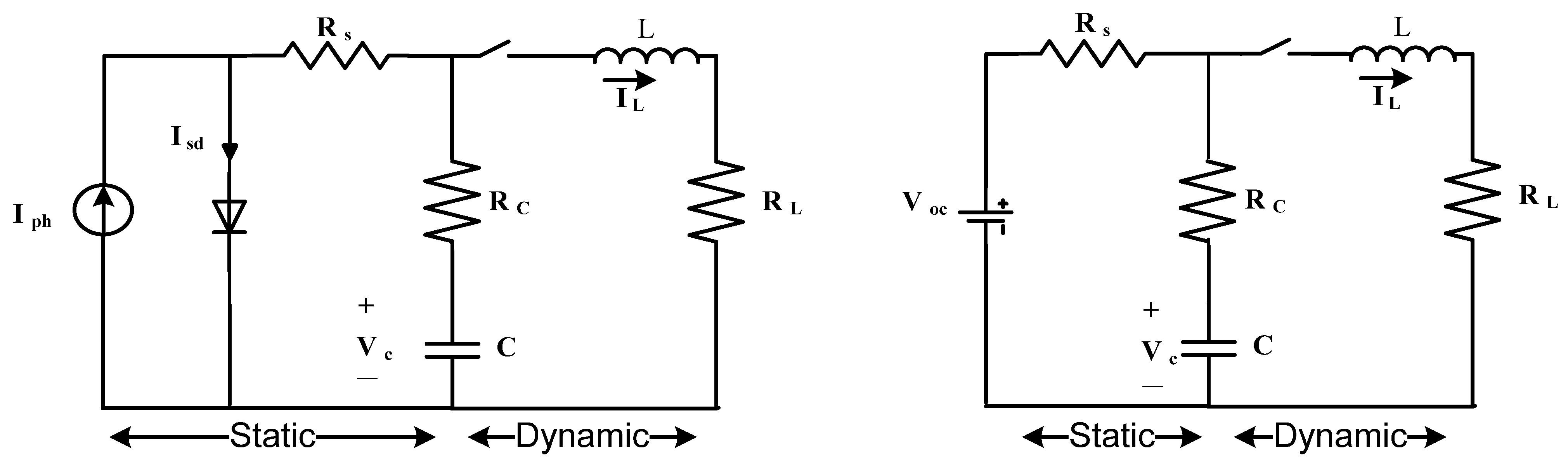Figure 4. Fractional Order dynamic model.
Figure 4. Fractional Order dynamic model.Figure 5. Flowchart of the proposed ESCGBO algorithm.
Figure 5. Flowchart of the proposed ESCGBO algorithm.Figure 6. Qualitative metrics on F1, F3, F4, F5, F8, F12, F15, and F18: 2D views of the functions, search history, average fitness history, and convergence curve using ESCGBO algorithm.
Figure 6. Qualitative metrics on F1, F3, F4, F5, F8, F12, F15, and F18: 2D views of the functions, search history, average fitness history, and convergence curve using ESCGBO algorithm.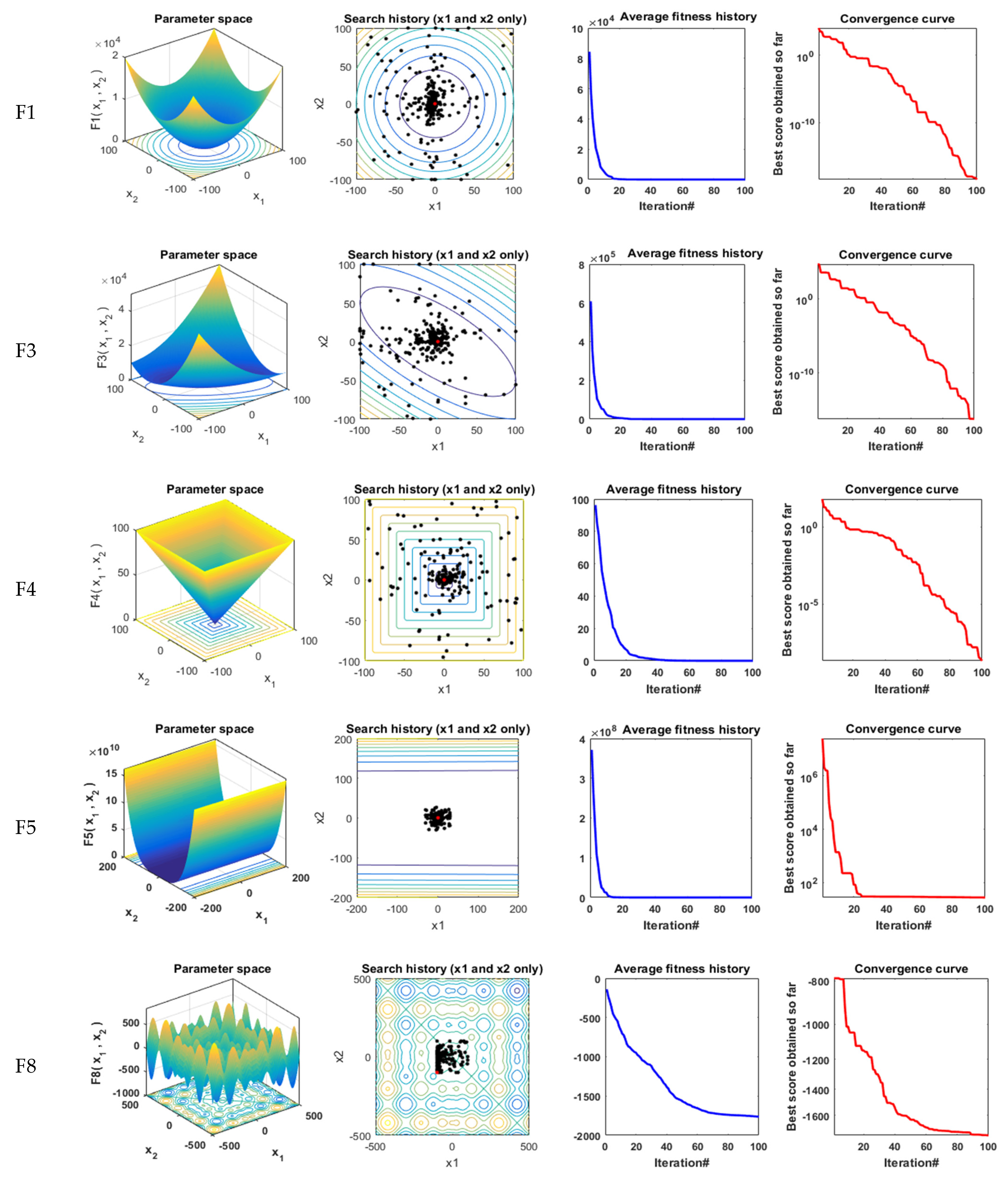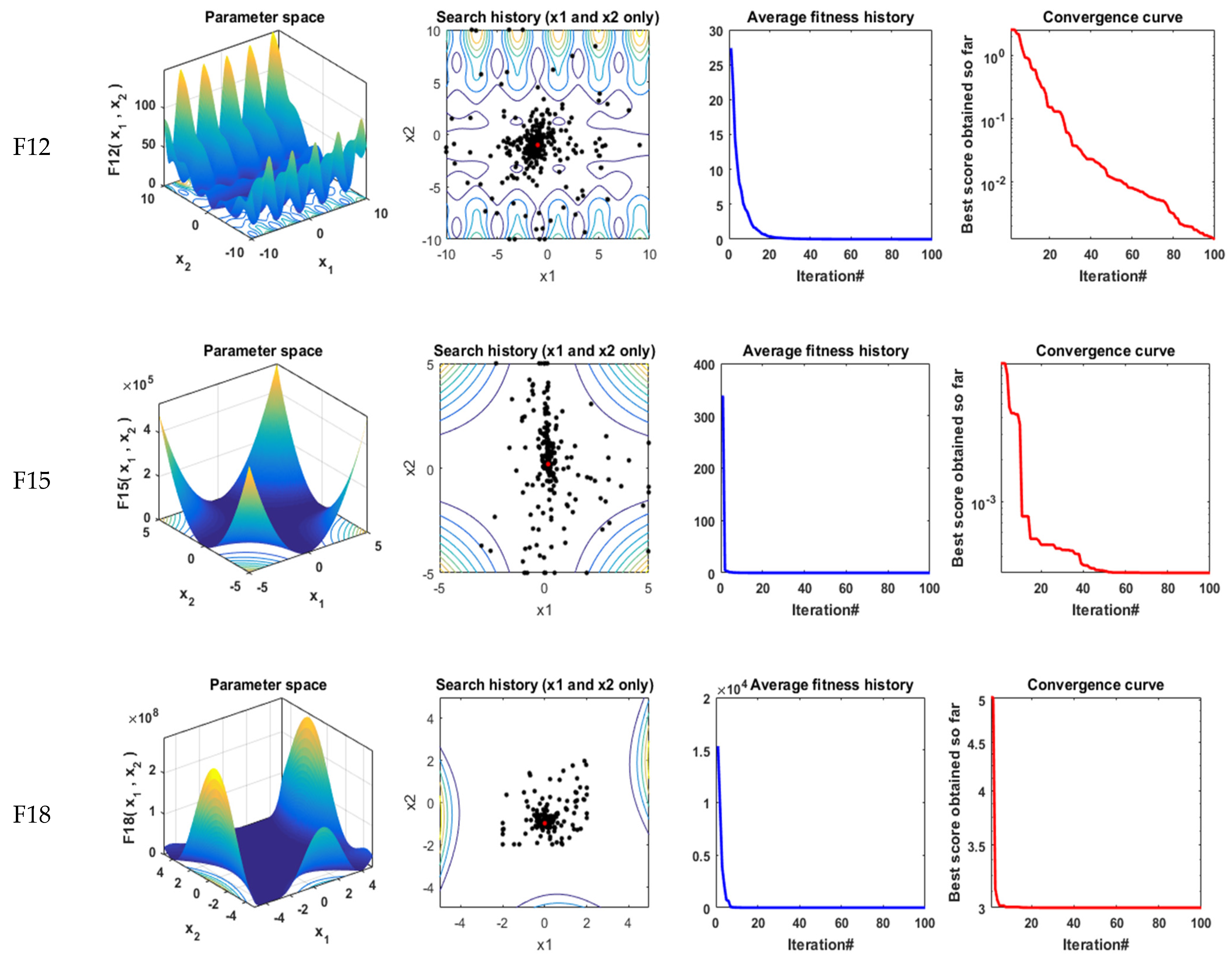Figure 7. The convergence curves of all algorithms for unimodal benchmark functions (a) F1, (b) F2, (c) F3, (d) F4, (e) F5, (f) F6, and (g) F7.
Figure 7. The convergence curves of all algorithms for unimodal benchmark functions (a) F1, (b) F2, (c) F3, (d) F4, (e) F5, (f) F6, and (g) F7.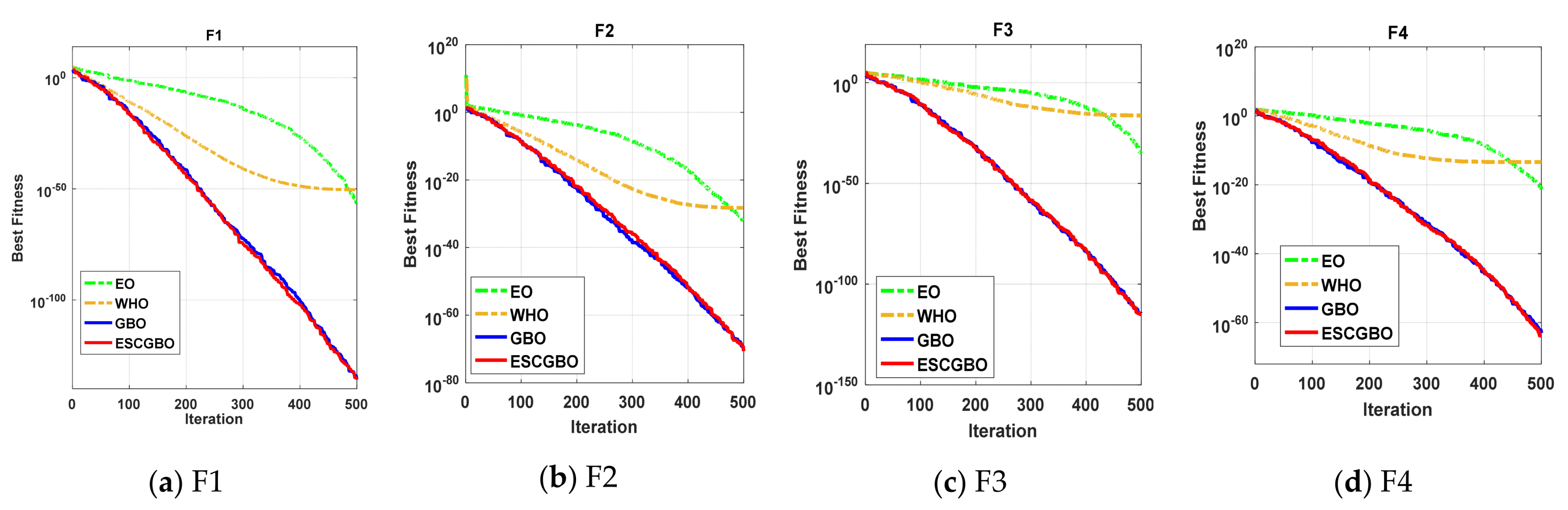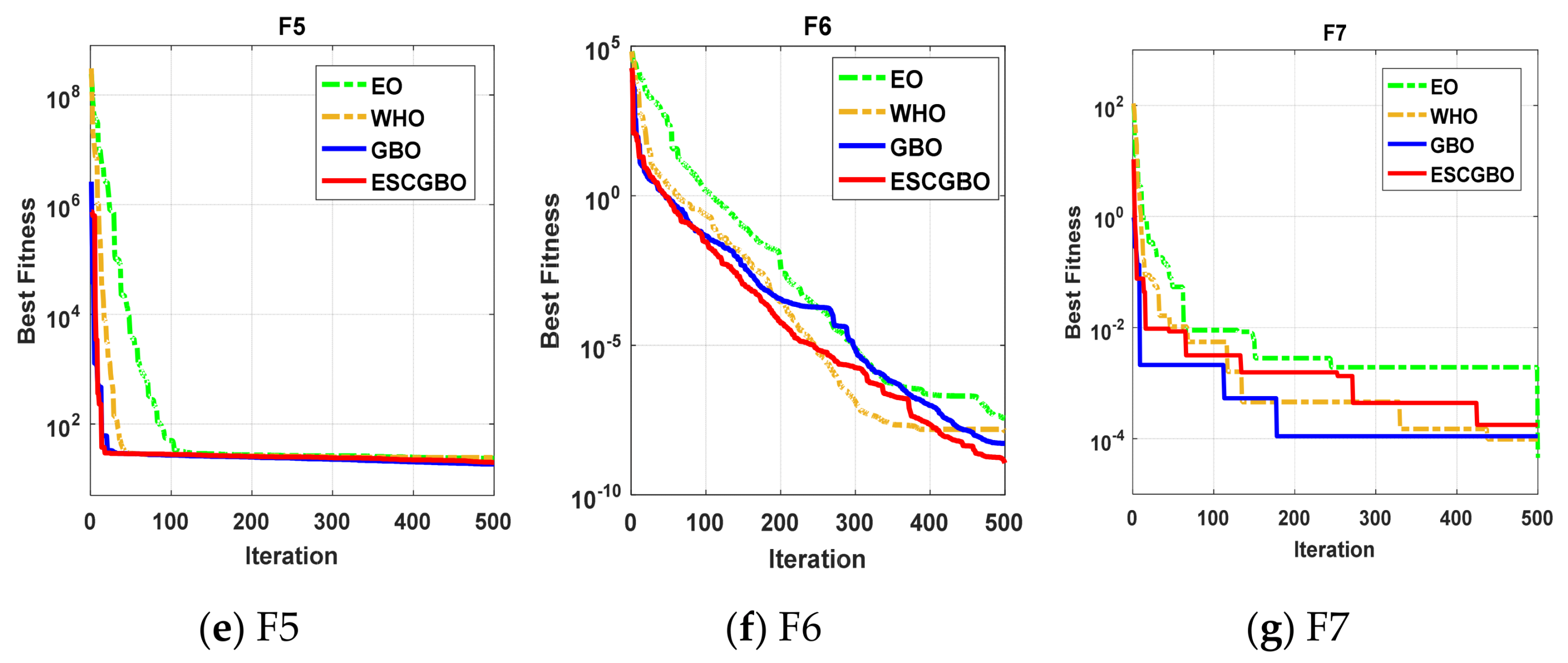Figure 8. Boxplots for all algorithms for unimodal benchmark functions (a) F1, (b) F2, (c) F3, (d) F4, (e) F5, (f) F6, and (g) F7.
Figure 8. Boxplots for all algorithms for unimodal benchmark functions (a) F1, (b) F2, (c) F3, (d) F4, (e) F5, (f) F6, and (g) F7.Figure 9. The convergence curves of all algorithms for multi-modal benchmark functions (a) F8, (b) F9, (c) F10, (d) F11, (e) F12, and (f) F13.
Figure 9. The convergence curves of all algorithms for multi-modal benchmark functions (a) F8, (b) F9, (c) F10, (d) F11, (e) F12, and (f) F13.Figure 10. Boxplots for all algorithms for some of multi-modal benchmark functions (a) F8, (b) F10, (c) F12, and (d) F13.
Figure 10. Boxplots for all algorithms for some of multi-modal benchmark functions (a) F8, (b) F10, (c) F12, and (d) F13.Figure 11. The convergence curves of all algorithms for composite benchmark functions (a) F14, (b) F15, (c) F16, (d) F17, (e) F18, (f) F19, (g) F20, (h) F21, (i) F22, and (j) F23.
Figure 11. The convergence curves of all algorithms for composite benchmark functions (a) F14, (b) F15, (c) F16, (d) F17, (e) F18, (f) F19, (g) F20, (h) F21, (i) F22, and (j) F23.Figure 12. Boxplots for all algorithms for some of composite benchmark functions (a) F14, (b) F15, (c) F20, (d) F21, (e) F22, and (f) F23.
Figure 12. Boxplots for all algorithms for some of composite benchmark functions (a) F14, (b) F15, (c) F20, (d) F21, (e) F22, and (f) F23.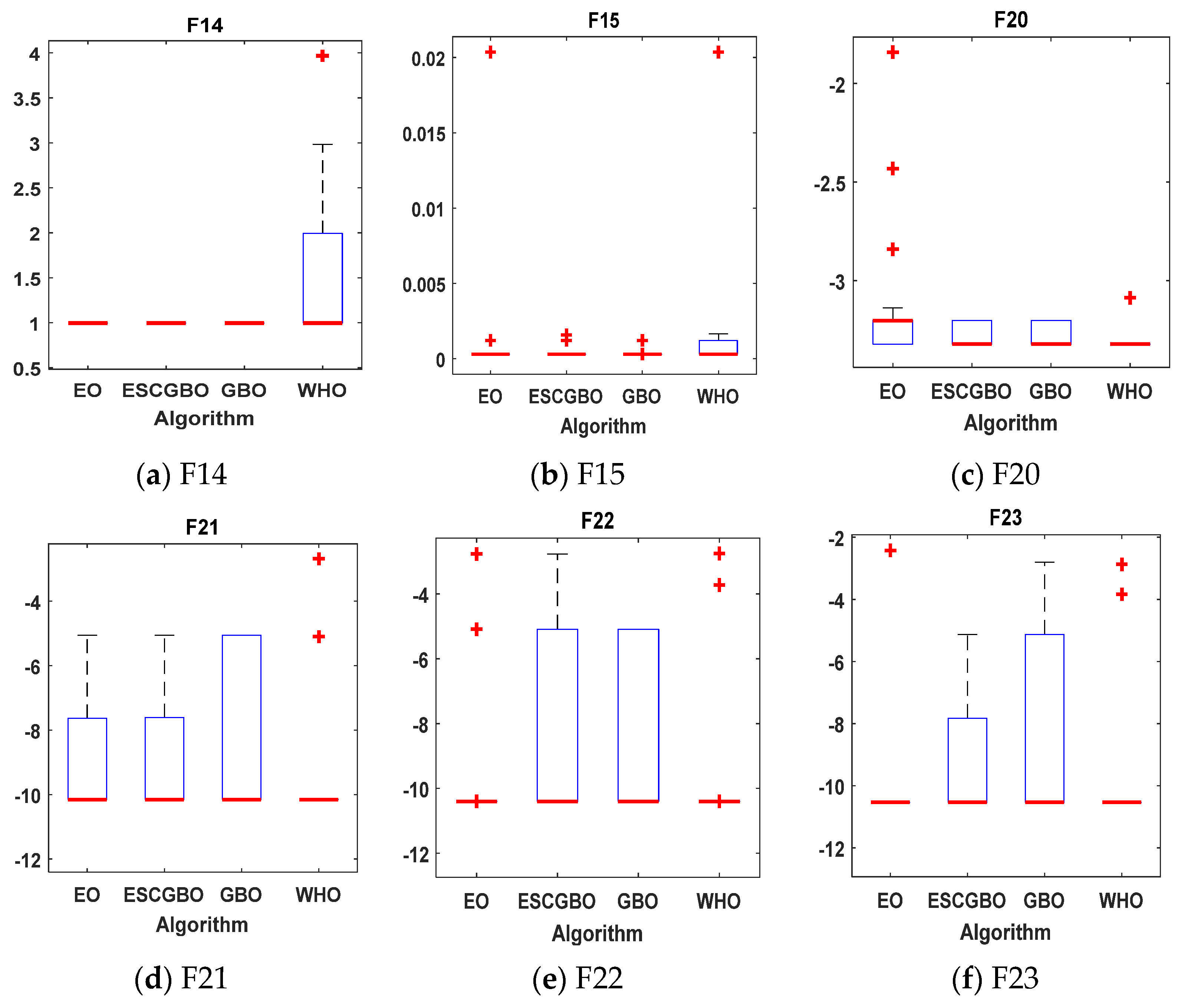Figure 13. The convergence curve of all algorithms of SDM.
Figure 13. The convergence curve of all algorithms of SDM.Figure 14. The convergence curve of all algorithms of DDM.
Figure 14. The convergence curve of all algorithms of DDM.Figure 15. Boxplot figure of all algorithms for 30 independent runs in case of SDM and DDM.
Figure 15. Boxplot figure of all algorithms for 30 independent runs in case of SDM and DDM.Figure 16. Power and current characteristics for SDM estimated by all algorithms.
Figure 16. Power and current characteristics for SDM estimated by all algorithms.Figure 17. Power and current characteristics for DDM estimated by all algorithms.
Figure 17. Power and current characteristics for DDM estimated by all algorithms.Figure 18. Power and current absolute error for SDM estimated by all algorithms.
Figure 18. Power and current absolute error for SDM estimated by all algorithms.Figure 19. Power and current absolute error for DDM estimated by all algorithms.
Figure 19. Power and current absolute error for DDM estimated by all algorithms.Figure 20. Convergence curves of all algorithms for IOM.
Figure 20. Convergence curves of all algorithms for IOM.Figure 21. Convergence curves of all algorithms for FOM.
Figure 21. Convergence curves of all algorithms for FOM.Figure 22. Load current curve of real data and the estimated IOM by different algorithms.
Figure 22. Load current curve of real data and the estimated IOM by different algorithms.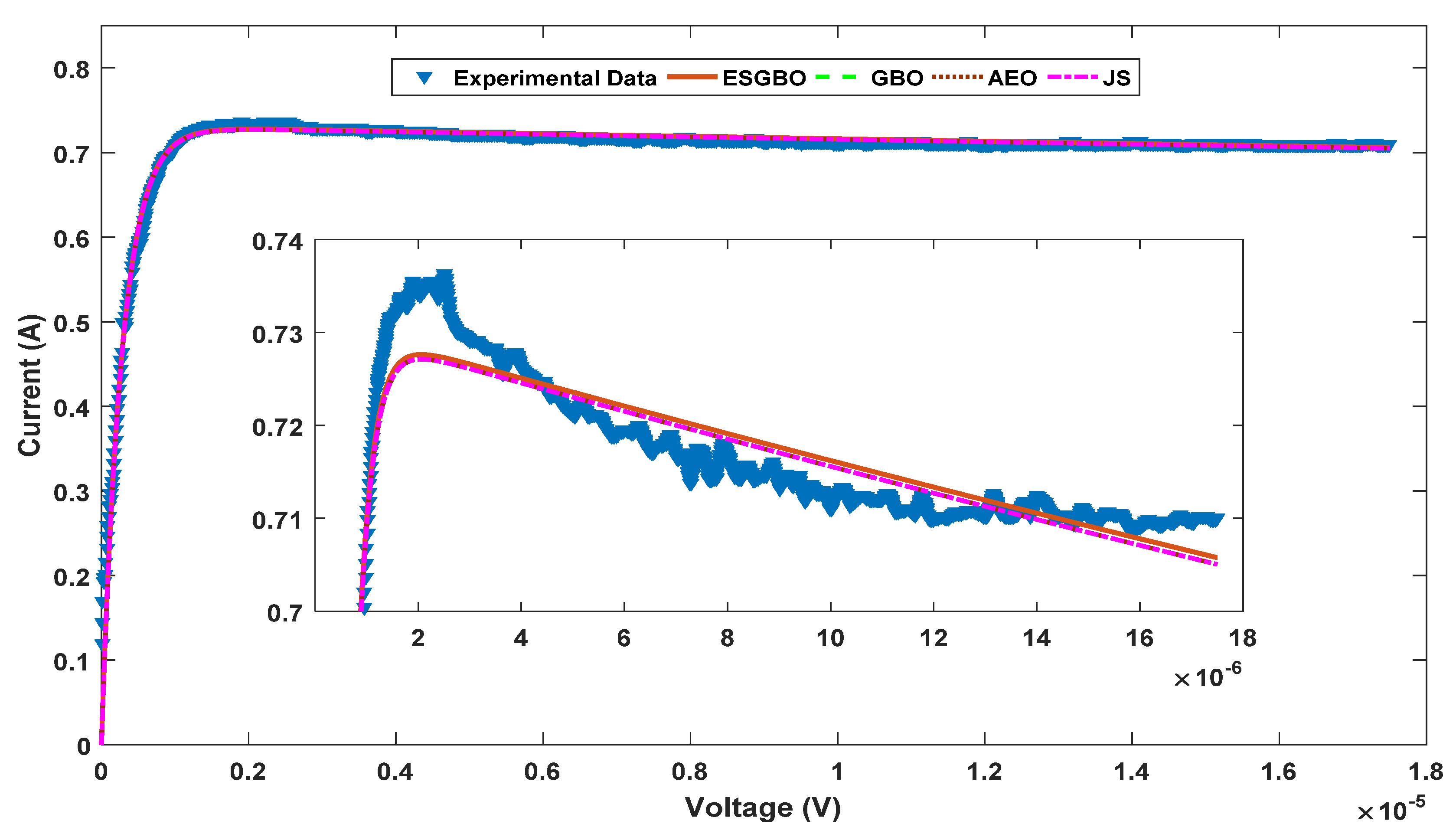Figure 23. Load current curve of real data and the estimated FOM by different algorithms.
Figure 23. Load current curve of real data and the estimated FOM by different algorithms.Figure 24. Current absolute error of the estimated IOM by different algorithms.
Figure 24. Current absolute error of the estimated IOM by different algorithms.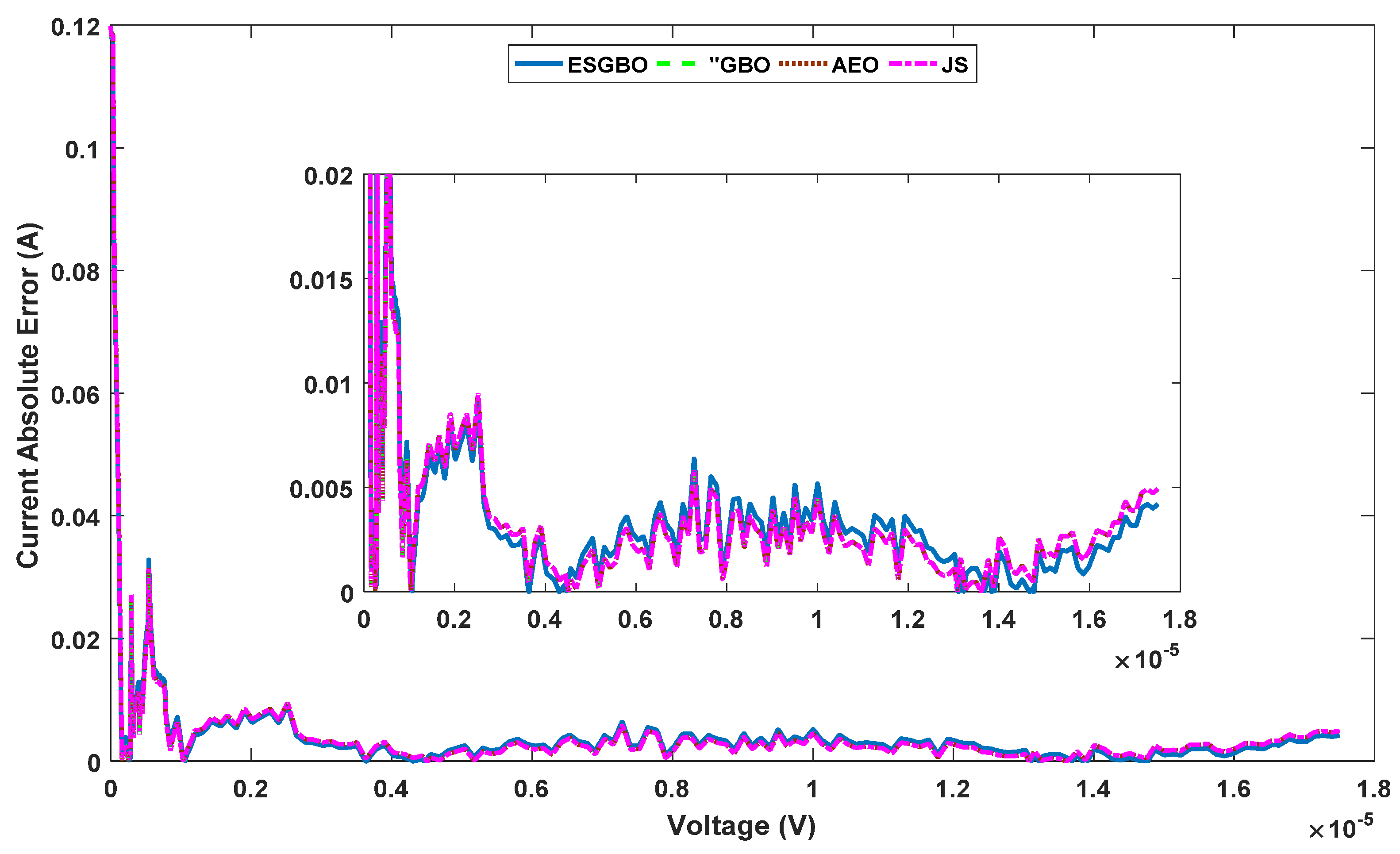Figure 25. Current absolute error of the estimated FOM by different algorithms.
Figure 25. Current absolute error of the estimated FOM by different algorithms.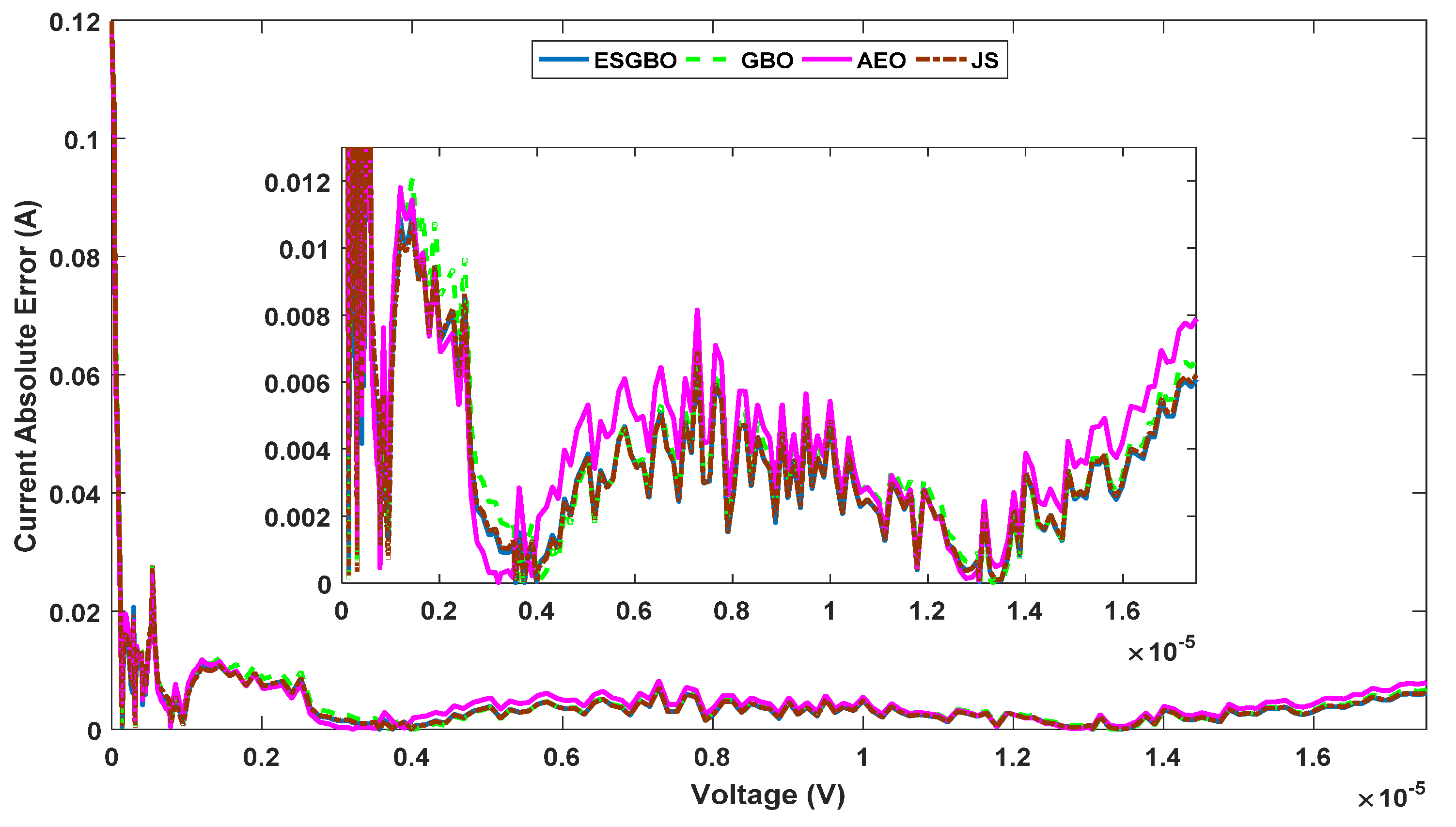Table 1. The statistical results of unimodal benchmark functions using the proposed technique and other well-known algorithms.
Table 1. The statistical results of unimodal benchmark functions using the proposed technique and other well-known algorithms.
FunctionESCGBOGBOWHOEO
F1Best2.20 × 10−1361.44 × 10−1362.83 × 10−573.89 × 10−51
Worst1.14 × 10−1296.32 × 10−1283.53 × 10−476.09 × 10−47
Mean1.33 × 10−1307.41 × 10−1291.78 × 10−484.11 × 10−48
std3.38 × 10−1301.86 × 10−1287.90 × 10−481.36 × 10−47
F2Best2.31 × 10−712.89 × 10−708.31 × 10−335.47 × 10−29
Worst2.33 × 10−651.84 × 10−669.82 × 10−291.3 × 10−27
Mean1.39 × 10−662.39 × 10−671.39 × 10−293.65 × 10−28
std5.2 × 10−664.92 × 10−672.48 × 10−293.47 × 10−28
F3Best8.7 × 10−1161.6 × 10−1156.42 × 10−365.41 × 10−17
Worst6.7 × 10−1043.9 × 10−1016.83 × 10−281.39 × 10−11
Mean3.4 × 10−1051.9 × 10−1025.22 × 10−292.34 × 10−12
std1.5 × 10−1048.7 × 10−1021.66 × 10−284.08 × 10−12
F4Best1.58 × 10−648.91 × 10−645.07 × 10−224 × 10−14
Worst3.03 × 10−581.63 × 10−593.71 × 10−196.87 × 10−12
Mean1.72 × 10−591.6 × 10−604.56 × 10−201.2 × 10−12
std6.75 × 10−593.65 × 10−609.74 × 10−201.77 × 10−12
F5Best19.9969818.5238823.6095524.44135
Worst25.0249124.0238986.1944425.0421
Mean21.6888821.2265834.3022824.81639
std1.3245681.16970520.977130.201379
F6Best1.14 × 10−95.23 × 10−93.67 × 10−81.39 × 10−8
Worst1.61 × 10−71.07 × 10−62.02 × 10−53.8 × 10−7
Mean3.35 × 10−87.89 × 10−81.42 × 10−69.07 × 10−8
std3.91 × 10−82.35 × 10−74.46 × 10−69.27 × 10−8
F7Best0.0001760.0001114.5 × 10−59.73 × 10−5
Worst0.0017320.0016630.001840.001432
Mean0.0005780.0005280.0008570.00068
std0.0004270.0003920.0005140.000358
The best values obtained are in bold.
Table 2. The statistical results of multimodal benchmark functions using the proposed technique and other well-known algorithms.
Table 2. The statistical results of multimodal benchmark functions using the proposed technique and other well-known algorithms.
FunctionESCGBOGBOWHOEO
F8Best−1881.33−1909.05−1789.02−1798.26
Worst−1668.99−1659.76−1600.49−1715.16
Mean−1733.03−1771.42−1705.23−1751.04
Std54.1839283.0158149.6229221.99425
F9Best0.000.000.000.00
Worst0.000.000.000.00
Mean0.000.000.000.00
Std0.000.000.000.00
F10Best8.88 × 10−168.88 × 10−168.88 × 10−1620
Worst8.88 × 10−168.88 × 10−1620.0011120
Mean8.88 × 10−168.88 × 10−161.00525920
Std0.000.004.4712211.23 × 10−10
F11Best0.000.000.000.00
Worst0.000.000.000.00
Mean0.000.000.000.00
Std0.000.000.000.00
F12Best9.51 × 10−121.45 × 10−101.18 × 10−111.42 × 10−10
Worst1.62 × 10−70.1036690.1036691.98 × 10−7
Mean9.05 × 10−90.0051830.015551.25 × 10−8
Std3.61 × 10−80.0231810.0379794.37 × 10−8
F13Best9.97 × 10−92.29 × 10−87.36 × 10−81.63 × 10−8
Worst0.0547790.0547790.3978010.108359
Mean0.010430.0163510.0358140.018527
Std0.0144440.0198720.0938810.037211
The best values obtained are in bold.
Table 3. The statistical Results of composite benchmark functions using the proposed technique and other well-known algorithms.
Table 3. The statistical Results of composite benchmark functions using the proposed technique and other well-known algorithms.
FunctionESCGBOGBOWHOEO
F14Best0.9980040.9980040.9980040.998004
Worst0.9980040.9980043.968250.998004
Mean0.9980040.9980041.5435340.998004
Std0.000.000.9362991.76 × 10−16
F15Best0.0003070.0003070.0003070.000307
Worst0.0015940.0012230.0203630.020363
Mean0.0005280.0004450.0016760.002359
Std0.000460.0003350.0044240.006161
F16Best−1.03163−1.03163−1.03163−1.03163
Worst−1.03163−1.03163−1.03163−1.03163
Mean−1.03163−1.03163−1.03163−1.03163
Std2.28 × 10−162.28 × 10−161.35 × 10−162.1 × 10−16
F17Best0.3978870.3978870.3978870.397887
Worst0.3978870.3978870.3978870.397887
Mean0.3978870.3978870.3978870.397887
Std0.000.000.000.00
F18Best3.003.003.003.00
Worst3.003.003.003.00
Mean3.003.003.003.00
Std8.7 × 10−164.2 × 10−165.85 × 10−166.76 × 10−16
F19Best−0.30048−0.30048−0.30048−0.30048
Worst−0.30048−0.30048−0.30048−0.30048
Mean−0.30048−0.30048−0.30048−0.30048
Std1.14 × 10−161.14 × 10−161.14 × 10−161.14 × 10−16
F20Best−3.322−3.322−3.322−3.322
Worst−3.2031−3.2031−3.08668−1.84092
Mean−3.28633−3.28633−3.31023−3.12852
Std0.0558990.0558990.0526190.370083
F21Best−10.1532−10.1532−10.1532−10.1532
Worst−5.0552−5.0552−2.68286−5.0552
Mean−8.8787−8.3689−9.52706−8.88098
Std2.2648462.4947611.9667232.260817
F22Best−10.4029−10.4029−10.4029−10.4029
Worst−2.7659−5.08767−2.75193−2.7659
Mean−7.82681−8.5426−8.96998−9.75532
Std2.9732542.6010822.9488312.02859
F23Best−10.5364−10.5364−10.5364−10.5364
Worst−5.12848−2.80663−2.87114−2.42173
Mean−9.18443−8.25715−9.148−10.1307
Std2.4025362.9070552.8553931.814497
The best values obtained are in bold.
Table 4. CPU time (s) of four algorithms on 23 benchmark functions.
Table 4. CPU time (s) of four algorithms on 23 benchmark functions.
ESCGBOGBOWHOEO
F12.241732.0452341.6667340.340013
F21.8316931.5499151.436070.3331
F32.6153741.9130631.6712680.365052
F41.9458161.7738112.094040.35823
F52.0326412.0283651.7040130.414698
F61.880231.6617281.6796130.326943
F72.0778341.6378861.4541670.371617
F81.7956021.5406291.4300210.341869
F91.8425141.6025241.6578410.3381
F101.7611221.5033071.3941210.35016
F111.7800451.6084811.643130.416369
F121.7463821.5053881.3667050.33033
F131.996151.8352351.430580.352283
F142.4178391.9524141.6087650.561999
F151.9858621.6828232.0375070.96656
F161.7549782.0520511.4071740.342419
F171.725061.4154341.5649040.375495
F182.7461231.6172942.49280.506535
F191.8915362.2002071.4706040.363025
F201.7929421.5094641.5325230.360518
F212.0098322.138491.564710.332705
F222.3302212.102972.3567060.375617
F232.1278422.0275981.5305250.360371
Table 5. Upper and lower constrains for all estimated parameters.
Table 5. Upper and lower constrains for all estimated parameters.
ParameterSolar Cell
Lower LimitUpper Limit
Rs05
Rsh0100
Iph02
Is101
Is201
ɳ 112
ɳ 212
Table 6. Parameters setting for all compared algorithms.
Table 6. Parameters setting for all compared algorithms.
AlgorithmControl Prameters
ESCGBOnP = 50pr = 0.5CM = 4
GBOnP = 50pr = 0.5CM = 4
AEOPopSize = 50r1 = rand
JSNpop = 50
WHON = 50PS = 0.2PC = 0.13
EOParticles_no = 50a1 = 2, a2 = 1GP = 0.5
Table 7. Estimated parameters and RMSE of ESCGBO and other algorithms for SDM model.
Table 7. Estimated parameters and RMSE of ESCGBO and other algorithms for SDM model.
ESCGBOGBOAEOJS
Rs (Ω)0.0363770.0363770.0363770910.035989122
Rsh (Ω)53.7185353.7185253.7185316457.40304457
Iph (A)0.7607760.7607760.760775530.760889273
Is (A)3.23 × 10−73.23 × 10−73.23 × 10−73.61 × 10−7
ɳ1.4768941.4768941.4768943141.48800365
RMSE0.0009860220.0009860220.0009860220.001025784
Table 8. Estimated parameters and RMSE of ESCGBO and other algorithms for DDM model.
Table 8. Estimated parameters and RMSE of ESCGBO and other algorithms for DDM model.
ESCGBOGBOAEOJS
Rs (Ω)0.0367426470.0363750730.0367069570.035338785
Rsh (Ω)55.5005676753.6889618955.3283569554.95529664
Iph (A)0.7607811680.760778920.7607832080.760597151
Is1 (A)7.64 × 10−73.78 × 10−92.34 × 10−73.51 × 10−7
Is2 (A)2.26 × 10−73.20 × 10−77.00 × 10−72.02 × 10−7
ɳ 11.999997561.4343766741.4495822571.487619855
ɳ 21.4468499341.4777244921.9999999841.916132114
RMSE0.0009824180.0009860290.0009824510.00119615
Table 9. The statistical results of SDM for all other algorithms.
Table 9. The statistical results of SDM for all other algorithms.
MinimumAverageMaximumSTD
ESCGBO0.0009860220.0009860260.0009860325.507 × 10−9
GBO0.0009860220.0009894550.0009960225.688 × 10−6
AEO0.0009860220.0009895550.0009960225.608 × 10−6
JS0.0010257840.0015257840.0020257840.0005
Table 10. The statistical results of DDM for all algorithms.
Table 10. The statistical results of DDM for all algorithms.
MinimumAverageMaximumSTD
ESCGBO0.0009824180.0009824540.0009825185.507 × 10−8
GBO0.0009860290.0009900320.0009970396.088 × 10−6
AEO0.0009824510.0009884510.0009924515.291 × 10−6
JS0.001106150.0014694830.002106150.00055
Table 11. Upper and lower constrains for all estimated parameters.
Table 11. Upper and lower constrains for all estimated parameters.
ParameterSolar Cell
Lower LimitUpper Limit
Rc020
C2 × 10−86 × 10−5
L5 × 10−6100 × 10−6
α0.81.1
β0.81.1
Table 12. Estimated parameters of IOM model for all algorithms.
Table 12. Estimated parameters of IOM model for all algorithms.
ESCGBOGBOAEOJS
Rc5.5835885.6247487535.6247486475.6247490
C8.30 × 10−68.16 × 10−68.16 × 10−68.15726 × 10−6
L7.43 × 10−67.47 × 10−67.47 × 10−67.47323 × 10−6
RMSE0.0082594440.0084930670.0084930670.008493067262
Table 13. Estimated parameters of FOM model for all algorithms.
Table 13. Estimated parameters of FOM model for all algorithms.
ESCGBOGBOAEOJS
Rc4.6171279165.0059842834.5502015394.698924713
C5.81 × 10−55.04 × 10−61.46 × 10−54.08 × 10−5
L1.50 × 10−51.35 × 10−51.73 × 10−51.44 × 10−5
A0.81.0261205350.9172306230.833404373
β0.9508407630.9571659250.9406545370.953192785
RMSE0.0079512890.0082360170.008195980.007995872
 Publisher’s Note: MDPI stays neutral with regard to jurisdictional claims in published maps and institutional affiliations.